## Tuesday, August 16, 2016

### Tinkering and Hammering Out Equations

This is how it's done.

So, you're wondering, "hey, exactly how are you going to crank out these equations people have been looking for for likely hundreds of years over the next few months?"  Well, just like I said way back in the beginning of the blog, we'll do it the old fashioned way, and that is step by step.

Alternative method:

The Surfer, OM-IV

## Monday, August 15, 2016

### We're Calculating Masses of Fundamental Particles Now!!!

We can now calculate, from first principles and fundamental Planck units, the mass of blackholes, protons, and electrons. (link to post about new electron holographic solution)

The proton actually is a fundamental particle as well.  The Standard Model considers that the proton is composed of quarks, thus not fundamental.

So, now, what about all of the other fundamental particles?  Can we also calculate their masses and predict all their masses correctly, and predict masses of other unknown particles?

For all the success of The Standard Model, it is incomplete, and, while it works very well for some things (QED, QCD, etc), it has issues.

Anyway, we can now calculate the mass of one of the elementary particles in the table above, the electron.  It seems then like the muon or tau particle would be the appropriate particle to target next to see if we can calculate its mass, and perhaps along the way determine or demonstrate exactly what works and what does not work with "The Standard Model" way of looking at things.

For now, we will list the masses of the first three leptons:
 electron mass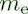Value 9.109 383 56 x 10-31 kgStandard uncertainty 0.000 000 11 x 10-31 kgRelative standard uncertainty 1.2 x 10-8Concise form 9.109 383 56(11) x 10-31 kg

 muon mass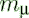Value 1.883 531 594 x 10-28 kgStandard uncertainty 0.000 000 048 x 10-28 kgRelative standard uncertainty 2.5 x 10-8Concise form 1.883 531 594(48) x 10-28 kg

 tau mass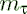Value 3.167 47 x 10-27 kgStandard uncertainty 0.000 29 x 10-27 kgRelative standard uncertainty 9.0 x 10-5Concise form 3.167 47(29) x 10-27 kg

And their relative mass ratios:

 muon-electron mass ratio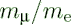Value 206.768 2826

 tau-electron mass ratio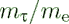Value 3477.15

 tau-muon mass ratio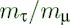Value 16.8167

Since the muon and tau are simply more energetic electrons, it seems like if these were actual particles, we should be able to find an equation that predicts their masses based upon first principles and fundamental Planck constants.

To do this, we will have to look more deeply into the muon and tau particles.  We are having great success so far at applying Haramein's Holographic Solution, and the approach will either solve for the masses of these other particles, or explain what is wrong with The Standard Model.

Since this work is too large to fit in the margin of this post, we'll do it over the next few posts, and months, until some resolution is made.

The writing is already on the wall that The Standard Model has missed the boat, while at the same time MISSING a whole lot of things that really matter.

That's all for now.

The Surfer, OM-IV

## Sunday, August 14, 2016

### Another Equation for Proton to Electron Mass Ratio???

$$\mu={m_p\over m_e}={2\phi m_{\ell}\over {\phi_em_{\ell}/2\alpha}}=4\alpha{\phi\over\phi_e}=4\alpha{a_0\over r_p}=1836.15267...$$
Compare to:
$$\mu={\alpha^2\over{\pi r_pR_{\infty}}}=1836.15267...$$
$\mu=$ proton to electron  mass ratio
$m_p=$ mass of proton
$m_e=$ mass of electron
$\phi={\eta\over R}$ Holographic ratio for proton
$\phi_e={\eta_e\over R_e}$ Holographic ratio for electron
$m_{\ell}=$ Planck mass
$a_0=$ Bohr radius
$r_p=$ proton radius (muonic hydrogen proton radius)
$R_{\infty}=$ Rydberg constant

((4 * fine-structure constant * hbar) / (m_e * c * fine-structure constant)) / (((4 * hbar) / c) / m_p) =
1 836.15267

CODATA value for proton-electron mass ratio:
http://physics.nist.gov/cgi-bin/cuu/Value?mpsme
 proton-electron mass ratio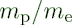Value 1836.152 673 89Standard uncertainty 0.000 000 17Relative standard uncertainty 9.5 x 10-11Concise form 1836.152 673 89(17)

There remain a few issues to be ironed out as Haramein's paper, The Electron and the Holographic Mass Solution, reports the proton to electron mass ratio as:
$$\mu={m_p\over m_e}={2\phi m_{\ell}\over {\phi_em_{\ell}/2\alpha}}=4\alpha{\phi\over\phi_e}=1836.942579077855...$$

So, the work continues to understand this difference...

This result, the 1836.94259077855... above, is calculated using the CODATA value for $\alpha$, the 2013 muonic hydrogen charge radius in the $phi$ calculation, and $phi_e=6.108458512E-25$ .  See Google Drive Excel File for calculations.

More in upcoming post: Another Equation for Proton to Electron Mass Ratio!!! #2

The Surfer, OM-IV

## Saturday, August 13, 2016

### The Electron and the Holographic Mass Solution #2

Sword of Science - SoS

...continuing with Haramein's The Electron and the Holographic Mass Solution:
Let's examine the expression for the electron mass and go through the exercise of how to put numbers to the equations and compare the results to CODATA measurements and previous mainstream theoretical predictions and analysis.
$$m_e={1 \over 2\alpha}\phi_e m_\ell$$
$m_e=$ mass of the electron
$m_\ell=$ Planck mass
$\phi_e={\eta_e\over R_e}=$ electron Holographic ratio
$\eta_e=$ Surface entropy
$R_e=$ Volume entropy
$\alpha=$ fine-structure constant

The subscript "e", for electron, is to emphasize that the $\eta_e$ and $R_e$ are to be calculated for the electron, using the confined electron's Bohr hydrogen radius atomic volume and the surface area to calculate $\eta$ and $R$.

Let's do $\eta$, the surface entropy first:
$$\eta=\eta_e$$
$$\eta_e={A_{e-surface}\over A_{eq}}$$
$$A_{e-surface}=4\pi {a_{0}}^2$$
$$A_{eq-PSU}=\pi {r_{\ell}}^2$$
$a_0=$ Bohr hydrogen radius - distance electron is away from proton center
$r_{\ell}$ is the Planck radius of the PSU
$r_{\ell}={\ell\over 2}$
$\ell=$ Planck length
$A_{e-surface}$ is the surface area of a sphere of radius $a_0$, the Bohr radius.
$A_{eq-PSU}$ is the equatorial cross-sectional area of the Planck Spherical Unit (PSU).

$$\eta=\eta_e={4\pi {a_{0}}^2\over \pi {r_{\ell}}^2}$$

$R_e$, the volume entropy is next:
$$R_e={V_e\over V_{PSU}}$$
$$R_e={{4\over3}\pi a_0^3\over {4\over3}\pi r_{\ell}^3}={a_0^3\over r_{\ell}^3}$$

And finally:
$$\phi_e={\eta_e\over R_e}$$
$$\phi_e={4r_{\ell}\over a_0}$$
$$m_e={{1\over2\alpha}\phi_em_{\ell}}$$

electron mass =
9.10938356 × 10-31 kilograms

CODATA Link to M_e electron mass
 electron massValue 9.109 383 56 x 10-31 kgStandard uncertainty 0.000 000 11 x 10-31 kgRelative standard uncertainty 1.2 x 10-8Concise form 9.109 383 56(11) x 10-31 kg

Check!!!  Another success of Haramein's holographic approach.

Some further simplifications/reductions:
$$m_e={{1\over2\alpha}{4r_{\ell}\over a_0}m_{\ell}}$$
$$m_e={\ell m_{\ell}\over\alpha a_0}$$
$$\ell=\sqrt{\hbar G \over c^3}$$
$$m_{\ell}=\sqrt{\hbar c\over G}$$
$${\ell}m_{\ell}={\hbar\over c}$$

$$m_e={\hbar\over c\alpha a_0}$$
$$a_0={\hbar\over m_ec\alpha}$$

The Surfer, OM-IV

## Friday, August 12, 2016

### The Revolution in Particle Physics continues!!!

It will be interesting to see how it all unfolds.  A few recent online articles mention a third verification of the proton radius problem, physics definately is in trouble:

Two areas will be opened by the unified physics:
1. Gravitational control (anti-grav)
2. ZPE, or energy extraction from the vacuum (and matter creation as well)
And look at all of the long unsolved physics problems and mysteries and paradoxes that are slowly, step by step, being explained by the unified physics of Haramein.

The Revolution in Particle Physics continues!!!
The Surfer, OM-IV

### Nassim Haramein: The Electron and the Holographic Mass Solution #1

Nassim Haramein and Amira K. F. Val Baker have written a paper that extends Haramein's holographic mass technique/approach to the electron.  This approach is derived from first principles and fundamental Planck units based on a geometric and informetric/entropic ratios.

From http://hiup.org/publications/: (link to Haramein's publication)

#### The Electron and the Holographic Mass Solution

Haramein, N & Val Baker, A. K. F. (2016). The Electron and the Holographic Mass Solution, ATINER 4th International Conference of Physics: Abstract Book, Page 14

Let's look at the black hole, proton, and the new electron mass equations, in general form before we break it down into specifics:
$$m_{BH}={m_\ell \over \phi}$$
$$m_p={2\phi m_\ell}$$
$$m_e={1 \over 2\alpha}\phi_e m_\ell$$
$m_{BH}=$ Schwarzschild mass of the black hole
$m_p=$ mass of the proton
$m_e=$ mass of the electron
$m_\ell=$ Planck mass
$\phi={\eta\over R}=$ Holographic ratio
$\phi_e={\eta\over R}=$ electron Holographic ratio
$\eta=$ Surface entropy
$R=$ Volume entropy
$\alpha=$ fine-structure constant
The general form for mass is:
$$mass=f(\phi)m_\ell$$
where we simply need to know the function of $\phi$ required for the object in question. Determining this function phi requires a little insight into the nature of the object and the geometric and informetric ratios.  This is an area we'll explore more later.
The solution for the electron proceeds exactly the same as for the black hole and the proton, basically calculating $\eta$ and $R$ using the Bohr radius, $a_0$, for the electron.  Details are in the paper linked above, and we will go over it more in later posts.
Significantly, in addition to the electron mass, Haramein has provided new, more accurate DERIVATIONS of the Rydberg constant, fine-structure constant, and proton to electron mass ratio equation and the derivation and his approach gives insight into the origin and nature of the constants and may even be able to address "discrepancies between experimental and theoretical values."
This cannot be emphasized enough how significant it is, the insight Haramein's analysis gives into these constants and the source and nature of mass of matter.
Over the next few posts we'll go into more detail, as this is enough for now.

The Revolution in Particle Physics continues!!!
The Surfer, OM-IV

## Saturday, August 6, 2016

### COLD FUSION DISCOVERY

FYI:  #ColdFusion #LENR is for real.  This "new" effect has been suppressed by the "mainstream".

The mainstream scientist can't explain how it works because it requires the new physics to understand how it works.  (Nassim Haramein's theory combined with Dan Winter's optimization).

This technology is useful, however, there other other ZPE devices possible in the framework of the new physics that the mainstream is ignoring.

(the new physics is almost the same as the old mainstream physics, it simply requires a few new basic "assumptions" that connect everything - the big and the small)

It is a #transmutation process #Alchemy is for real. #Newton.

# SPECIAL REPORT: GOV'T HIDING COLD FUSION DISCOVERY

For a mainstream scientist to quickly see how the "threshold" of the reaction can be attained, consider phonons, phononic vibrations shaking the matrix, and the density of the matrix is the aether, with a density of the Planck mass to the Planck Spherical Units volume (PSU) which is VERY HIGH DENSITY of ENERGY per volume, so a little shaking goes along way interacting with the catalytic like doping layers that assist the Hydrogen transmutation to He, as well as other transmutations.

It's ideas like this that power James Gilliland's ships.

The Surfer, OM-IV